SSC CGL Previous Year Questions: Profit & Loss - 1

# SSC CGL Previous Year Questions: Profit & Loss - 1 - SSC CGL

Test Description

## 20 Questions MCQ Test SSC CGL (Tier - 1) - Previous Year Papers (Topic Wise) - SSC CGL Previous Year Questions: Profit & Loss - 1

SSC CGL Previous Year Questions: Profit & Loss - 1 for SSC CGL 2023 is part of SSC CGL (Tier - 1) - Previous Year Papers (Topic Wise) preparation. The SSC CGL Previous Year Questions: Profit & Loss - 1 questions and answers have been prepared according to the SSC CGL exam syllabus.The SSC CGL Previous Year Questions: Profit & Loss - 1 MCQs are made for SSC CGL 2023 Exam. Find important definitions, questions, notes, meanings, examples, exercises, MCQs and online tests for SSC CGL Previous Year Questions: Profit & Loss - 1 below.
Solutions of SSC CGL Previous Year Questions: Profit & Loss - 1 questions in English are available as part of our SSC CGL (Tier - 1) - Previous Year Papers (Topic Wise) for SSC CGL & SSC CGL Previous Year Questions: Profit & Loss - 1 solutions in Hindi for SSC CGL (Tier - 1) - Previous Year Papers (Topic Wise) course. Download more important topics, notes, lectures and mock test series for SSC CGL Exam by signing up for free. Attempt SSC CGL Previous Year Questions: Profit & Loss - 1 | 20 questions in 20 minutes | Mock test for SSC CGL preparation | Free important questions MCQ to study SSC CGL (Tier - 1) - Previous Year Papers (Topic Wise) for SSC CGL Exam | Download free PDF with solutions
 1 Crore+ students have signed up on EduRev. Have you?
SSC CGL Previous Year Questions: Profit & Loss - 1 - Question 1

### The profit earned on selling an article at ₹ 720 is half of the loss incurred on selling the same article at ₹ 360. What is the cost price of the article.    (SSC MTS 2018)

Detailed Solution for SSC CGL Previous Year Questions: Profit & Loss - 1 - Question 1

Let cost price be x
Profit = 720 – x
Loss = x – 360
According to the question,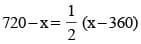1440 – 2x = x – 360
3x = 1800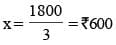SSC CGL Previous Year Questions: Profit & Loss - 1 - Question 2

### The ratio of the selling price to the cost price in a transaction is 4 : 5. If the selling price is ₹ 80, then how much is the loss?    (SSC MTS 2018)

Detailed Solution for SSC CGL Previous Year Questions: Profit & Loss - 1 - Question 2

Ratio of the selling price to the cost price is = 4 : 5
Selling price is ₹ 80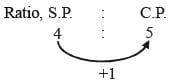Hence the loss is 1 unit
4 unit → 80
1 unit → 20
Hence the loss is 1 × 20 = ₹ 20

SSC CGL Previous Year Questions: Profit & Loss - 1 - Question 3

### Raman and Sanjay started a business by investing ₹ 63000 and ₹ 42000 respectively. If the total profit at the end of year is ₹ 9000, then what is the share of Raman?    (SSC MTS 2018)

Detailed Solution for SSC CGL Previous Year Questions: Profit & Loss - 1 - Question 3

Raman's capital = ₹ 63000
Sanjay 's capital = ₹ 42000
Total profit = ₹ 9000
as we know,
Capital × Time = Profit
So, Ratio of their investments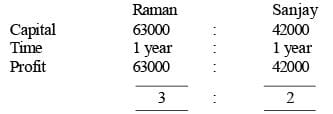Hence the Raman's share is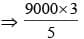⇒ 1800 × 3 ⇒ ₹ 5400

SSC CGL Previous Year Questions: Profit & Loss - 1 - Question 4

The marked price of an article is ₹ 3040. If the discount offered on this article is 20%, then what will be the selling price?    (SSC MTS 2018)

Detailed Solution for SSC CGL Previous Year Questions: Profit & Loss - 1 - Question 4

Marked price of an article is = 3040
Discount = 20% = 20/100 =  1/5
So, Hence the selling price = 4 unit.
Marked price is 5 unit.
5 unit → 3040
1 unit → 608
Hence the selling price is = 4 × 608 = ₹ 2432.

SSC CGL Previous Year Questions: Profit & Loss - 1 - Question 5

Two articles are sold for ₹ 962 each. On one, the seller gains 30% and on the other he loses 26%. What is his overall gain or loss percentage, nearest to one decimal place?    (SSC CGL-2018)

Detailed Solution for SSC CGL Previous Year Questions: Profit & Loss - 1 - Question 5

Cost price of one article =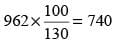Cost price of other article =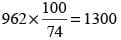Loss = (740 + 1300) – (962 + 962) = 116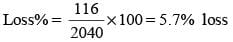SSC CGL Previous Year Questions: Profit & Loss - 1 - Question 6

Two articles are sold for ₹ 9,720 each. On one, the seller gains 8% and on the other, he loses 10%. What is his overall gain or loss?    (SSC CGL-2018)

Detailed Solution for SSC CGL Previous Year Questions: Profit & Loss - 1 - Question 6

Cost price of one article =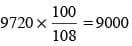Cost price of other article =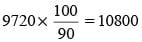Total loss = + Cost Price – Selling Price
= + 9000 + 10800 – 2 x 9720
= ₹ 360

SSC CGL Previous Year Questions: Profit & Loss - 1 - Question 7

A shopkeeper bought an article for ₹ 100 and marked its price 25% above the cost price. How much discount percentage should he announce in order to make a profit of 15%?    (SSC CHSL-2018)

Detailed Solution for SSC CGL Previous Year Questions: Profit & Loss - 1 - Question 7

Marked price of the article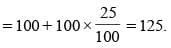selling price to get 15 % profit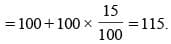Let discount percent is x%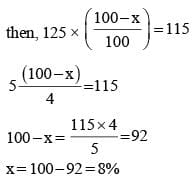SSC CGL Previous Year Questions: Profit & Loss - 1 - Question 8

A shopkeeper sold two articles for ₹ 9831 each. On one he gained 13% and on the other, he lost 13%. What is the 43 overall percentage gain or loss?    (SSC Sub. Ins. 2018 )

Detailed Solution for SSC CGL Previous Year Questions: Profit & Loss - 1 - Question 8

Cost price of first article =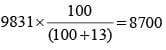On which gain is 13%
Cost price of second article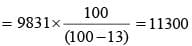Total cost price of two article = 8700 + 11300 = 20000
Total selling price of two article = 9831 + 9831 = 19662
Overall loss = 20000 – 19662 = 338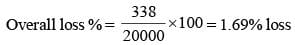SSC CGL Previous Year Questions: Profit & Loss - 1 - Question 9

A shopkeeper marks his good at a price such that after giving a discount of 25%, the gains 20%. If the marked price of the article is ₹ 736, what is the cost price of the article?    (SSC Sub-Inspector-2018 )

Detailed Solution for SSC CGL Previous Year Questions: Profit & Loss - 1 - Question 9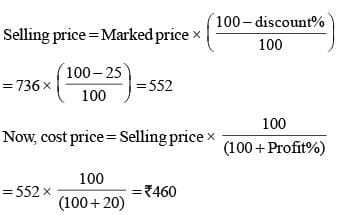SSC CGL Previous Year Questions: Profit & Loss - 1 - Question 10

A, B and C started a business by investing ₹ 55,000, ₹ 65,000 and ₹ 75,000 respectively. A is a working partner and gets 20% of the profit as working allowance and remaining is distributed in the proportion of their investment. If the money received by C is ₹ 27,000 what is the total profit?    (SSC Sub-Inspector-2018)

Detailed Solution for SSC CGL Previous Year Questions: Profit & Loss - 1 - Question 10

Ratio of investment of A, B and C
= 55000 : 65000 : 75000 = 11 : 13 : 15.
Let the total profit is x.
then, profit amount that has to be distributed among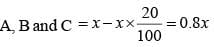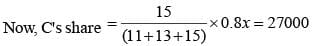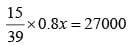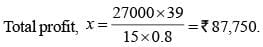SSC CGL Previous Year Questions: Profit & Loss - 1 - Question 11

By selling a fan for ₹ 1900 a man has a loss of 5 %, then at what price (in ₹) should he sell the fan to gain 20%?    (SSC Sub. Ins. 2017)

Detailed Solution for SSC CGL Previous Year Questions: Profit & Loss - 1 - Question 11

SP of a fan = ₹ 1900
Loss = 5%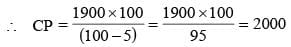Now,
Gain = 20%.
Then,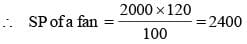∴ Selling price of a fan = ₹ 2400.

SSC CGL Previous Year Questions: Profit & Loss - 1 - Question 12

A man bought 15 mangoes for a rupee. How many mangoes were sold for a rupee so that there is a loss of 25%?    (SSC Sub. Ins. 2017)

Detailed Solution for SSC CGL Previous Year Questions: Profit & Loss - 1 - Question 12

CP of a man go = 1/15
Loss = 25%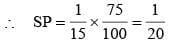So, A man sold 20 mangoes for a rupee.

SSC CGL Previous Year Questions: Profit & Loss - 1 - Question 13

An article is sold for ₹ 6552 after a discount of 22%. What is the marked price (in ₹) of the article?    (SSC Sub. Ins. 2017)

Detailed Solution for SSC CGL Previous Year Questions: Profit & Loss - 1 - Question 13

Let mark price be x.
According to question,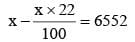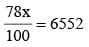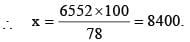∴ Marked price of the article = ₹ 8400.

SSC CGL Previous Year Questions: Profit & Loss - 1 - Question 14

At what percent above the cost price must a person mark the price of an article so that he can enjoy 20% profit after allowing 20% discount?    (SSC MTS 2017)

Detailed Solution for SSC CGL Previous Year Questions: Profit & Loss - 1 - Question 14

Let C.P = ₹ 100
Then S.P = ₹ 120
Let marked price be x
Then, 80% of x  = 120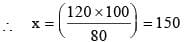∴ Marked price = 50% above C.P.

SSC CGL Previous Year Questions: Profit & Loss - 1 - Question 15

A shopkeeper sold a TV set for ₹ 17940 with a discount of 8% and earned a profit of 19.6%. What should have been the percentage of profit earned if no discount was offered?    (SSC MTS 2017)

Detailed Solution for SSC CGL Previous Year Questions: Profit & Loss - 1 - Question 15

According to question,
Selling price of the TV set = ₹ 17940
% discount = 8% and % gain = 19.6%
Let the cost price be ₹ 100
Gain = ₹ 19.6
∴ SP = 100 + 19.6 = 119.6
If SP is 119.6, CP = 100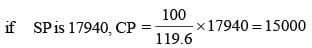Now, % discount = 8%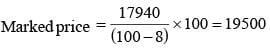if no discount is given, the selling price = 19500
∴ Profit = 19500 – 15000 = 4500
Therefore,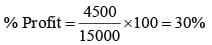So, the gain percent is = 30%

SSC CGL Previous Year Questions: Profit & Loss - 1 - Question 16

A fruit seller buys 100 kg of superior variety of mangoes at ₹ 45 per kg and 200 kgs of inferior variety at ₹ 40 per kg and sells all the mangoes at ₹ 45 per kg. The profit percentage of the fruit seller is:    (SSC MTS 2017)

Detailed Solution for SSC CGL Previous Year Questions: Profit & Loss - 1 - Question 16

Total CP of the mangoes = (100 × 45) + (200 × 40)
= 4500 + 8000 = 12500
Total SP of the mangoes = (300 × 45) = 13500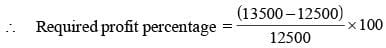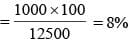SSC CGL Previous Year Questions: Profit & Loss - 1 - Question 17

A fan is listed at ₹ 150/- with a discount of 20%. What additional discount must be offered to the customer to bring the net price to ₹ 108/-?    (SSC MTS 2017)

Detailed Solution for SSC CGL Previous Year Questions: Profit & Loss - 1 - Question 17

M.P. of a fan = 150
Discount = 20%
Price of a fan after 20% discount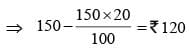Then,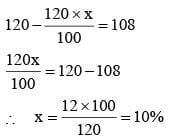SSC CGL Previous Year Questions: Profit & Loss - 1 - Question 18

A man sells an article at a loss of 10%. If he had sold it for ₹ 75 more he would have gained 20%. The cost price of the article is (in ₹):    (SSC MTS 2017)

Detailed Solution for SSC CGL Previous Year Questions: Profit & Loss - 1 - Question 18

Let CP of an article = x
According to question,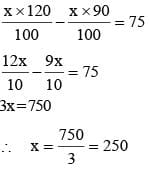∴ The cost price of an article = ₹ 250.

SSC CGL Previous Year Questions: Profit & Loss - 1 - Question 19

A merchant buys 20 kgs of a variety of rice at ₹ 14 per kg and another 40 kgs of rice at ₹ 10 per kg. He mixes them and sells 1/3 of the mixture at ₹ 12.50 per kg. At what rate should be sell the remaining mixture so as to earn a profit of 25% on the whole outlay?    (SSC MTS 2017)

Detailed Solution for SSC CGL Previous Year Questions: Profit & Loss - 1 - Question 19

CP = (20 × 14 + 40 × 10)
⇒ (280 + 400) = 680
Profit = 25%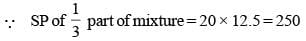∴ SP of remaining mixture = (850 – 250) = 600
∴ SP of remaining mixture per kg 600/40 = 15 per kg.

SSC CGL Previous Year Questions: Profit & Loss - 1 - Question 20

A shopkeeper by selling 13 Titan watches, earns a profit equal to the selling price of 3 Titan watches. His profit percentage is     (SSC CHSL 2017)

Detailed Solution for SSC CGL Previous Year Questions: Profit & Loss - 1 - Question 20

Let selling price of each watches = ₹ 1
∴ Selling price of 13 watches = 13
∵ Profit = 3 × selling price of watches = 3 × 1 = 3.
∴ Cost price of 13 wateres = (13 – 3) = 10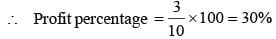## SSC CGL (Tier - 1) - Previous Year Papers (Topic Wise)

250 tests
Information about SSC CGL Previous Year Questions: Profit & Loss - 1 Page
In this test you can find the Exam questions for SSC CGL Previous Year Questions: Profit & Loss - 1 solved & explained in the simplest way possible. Besides giving Questions and answers for SSC CGL Previous Year Questions: Profit & Loss - 1, EduRev gives you an ample number of Online tests for practice

250 tests